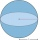# Sphere + third power - math problems

#### Number of problems found: 18

• Sphere growthHow many times grow volume of sphere if diameter rises 10×?
• Sphere fallHow many percent fall volume of a sphere if diameter decrease 3×?
• Cube in ballCube is inscribed into the sphere of radius 181 dm. How many percent is the volume of cube of the volume of the sphere?
• Sphere A2VThe surface of the sphere is 241 mm2. What is its volume?
• Hollow sphereCalculate the weight of a hollow stříbrné sphere (density 10.5 g/cm3) if the vnitřní diameter is 15 cm and wall thickness is 1 mm.
• Hollow sphereThe steel hollow sphere floats on the water plunged into half its volume. Determine the outer radius of the sphere and wall thickness, if you know that the weight of the sphere is 0.5 kg and the density of steel is 7850 kg/m3
• Iron sphereIron sphere has weight 100 kg and density ρ = 7600 kg/m3. Calculate the volume, surface and diameter of the sphere.
• SphereThe surface of the sphere is 12100 cm2, and the weight is 136 kg. What is its density?
• Chemical parisonThe blown parison (with shape of a sphere) have a volume 1.5 liters. What is its surface?
• The ballThe ball has a radius of 2m. What percentage of the surface and volume is another sphere whose radius is 20% larger?
• Alien shipThe alien ship has the shape of a sphere with a radius of r = 3000m, and its crew needs the ship to carry the collected research material in a cuboid box with a square base. Determine the length of the base and (and height h) so that the box has the large
• Volume of ballFind the volume of a volleyball that has a radius of 4 1/2 decimeters. Use 22/7 for π
• Find x 2Find x, y, and z such that x³+y³+z³=k, for each k from 1 to 100. Write down the number of solutions.
• BallsThree metal balls with volumes V1=71 cm3 V2=78 cm3 and V3=64 cm3 melted into one ball. Determine it's surface area.
• EightEight small Christmas balls with a radius of 1 cm have the same volume as one large Christmas ball. What has a bigger surface: eight small balls, or one big ball?
• Wall thicknessThe hollow metal ball has an outside diameter of 40 cm. Determine the wall thickness if the weight is 25 kg and the metal density is 8.45 g/cm3.
• Spherical segmentCalculate the volume of a spherical segment 18 cm high. The diameter of the lower base is 80 cm, the upper base 60 cm.
• Plasticine ballPlasticine balls have radius r1=85 cm, r2=60 mm, r3=59 cm, r4=86 cm, r5=20 cm, r6=76 mm, r7=81 mm, r8=25 mm, r9=19 mm, r10=14 cm. For these balls

Do you have an interesting mathematical word problem that you can't solve it? Submit a math problem, and we can try to solve it.

We will send a solution to your e-mail address. Solved examples are also published here. Please enter the e-mail correctly and check whether you don't have a full mailbox.

Please do not submit problems from current active competitions such as Mathematical Olympiad, correspondence seminars etc...

Sphere Problems. Third power - math word problems.